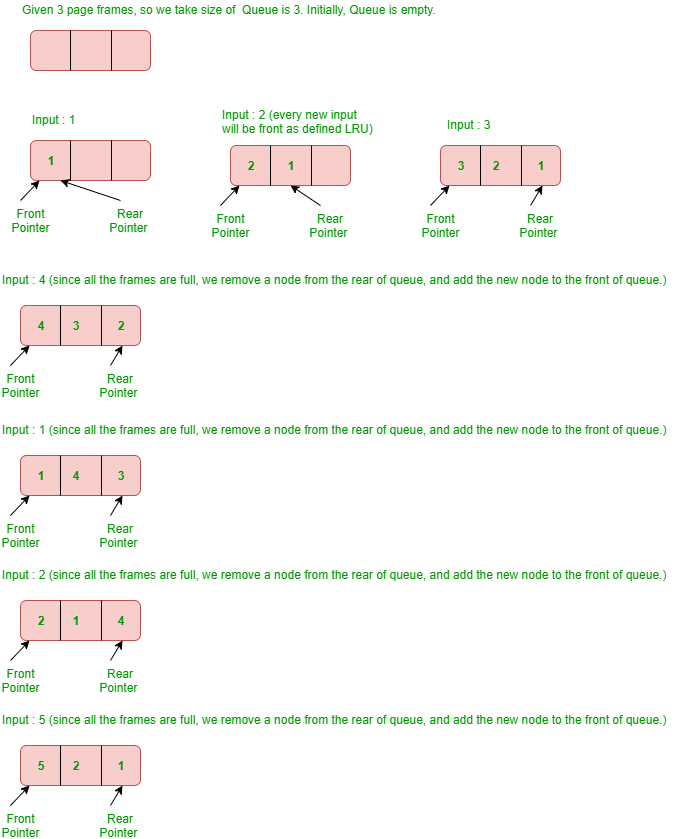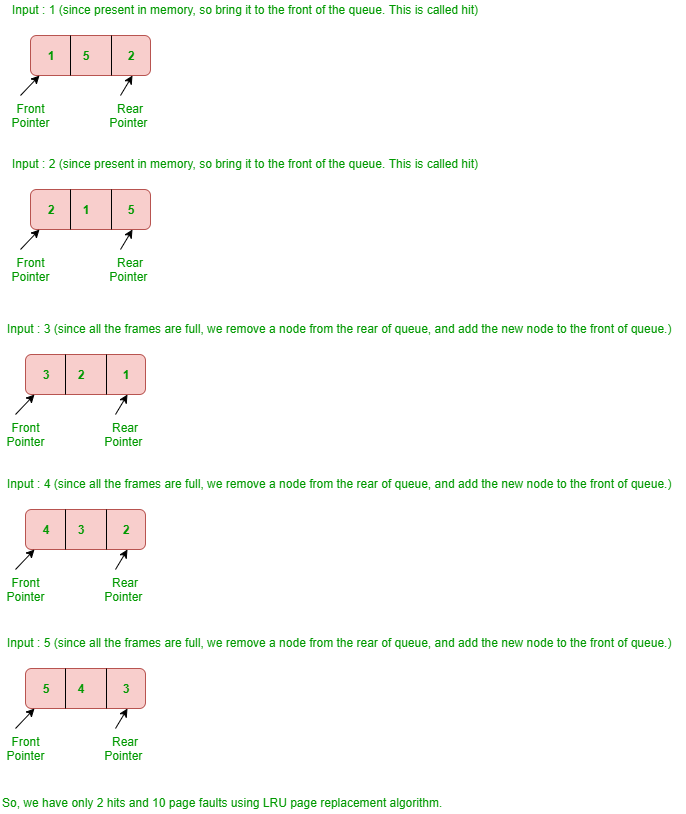Related Articles
LRU Cache Implementation
• Difficulty Level : Hard

How to implement LRU caching scheme? What data structures should be used?
We are given total possible page numbers that can be referred. We are also given cache (or memory) size (Number of page frames that cache can hold at a time). The LRU caching scheme is to remove the least recently used frame when the cache is full and a new page is referenced which is not there in cache. Please see the Galvin book for more details (see the LRU page replacement slide here).

We use two data structures to implement an LRU Cache.

1. Queue which is implemented using a doubly linked list. The maximum size of the queue will be equal to the total number of frames available (cache size). The most recently used pages will be near front end and least recently pages will be near the rear end.

2. A Hash with page number as key and address of the corresponding queue node as value.

When a page is referenced, the required page may be in the memory. If it is in the memory, we need to detach the node of the list and bring it to the front of the queue.
If the required page is not in memory, we bring that in memory. In simple words, we add a new node to the front of the queue and update the corresponding node address in the hash. If the queue is full, i.e. all the frames are full, we remove a node from the rear of the queue, and add the new node to the front of the queue.
Example – Consider the following reference string :

```1, 2, 3, 4, 1, 2, 5, 1, 2, 3, 4, 5
```

Find the number of page faults using least recently used (LRU) page replacement algorithm with 3 page frames.
Explanation –Note: Initially no page is in the memory.

## C++ using STL

 `// We can use stl container list as a double``// ended queue to store the cache keys, with``// the descending time of reference from front``// to back and a set container to check presence``// of a key. But to fetch the address of the key``// in the list using find(), it takes O(N) time.``// This can be optimized by storing a reference``//     (iterator) to each key in a hash map.``#include ``using` `namespace` `std;`` ` `class` `LRUCache {``    ``// store keys of cache``    ``list<``int``> dq;`` ` `    ``// store references of key in cache``    ``unordered_map<``int``, list<``int``>::iterator> ma;``    ``int` `csize; ``// maximum capacity of cache`` ` `public``:``    ``LRUCache(``int``);``    ``void` `refer(``int``);``    ``void` `display();``};`` ` `// Declare the size``LRUCache::LRUCache(``int` `n)``{``    ``csize = n;``}`` ` `// Refers key x with in the LRU cache``void` `LRUCache::refer(``int` `x)``{``    ``// not present in cache``    ``if` `(ma.find(x) == ma.end()) {``        ``// cache is full``        ``if` `(dq.size() == csize) {``            ``// delete least recently used element``            ``int` `last = dq.back();`` ` `            ``// Pops the last elmeent``            ``dq.pop_back();`` ` `            ``// Erase the last``            ``ma.erase(last);``        ``}``    ``}`` ` `    ``// present in cache``    ``else``        ``dq.erase(ma[x]);`` ` `    ``// update reference``    ``dq.push_front(x);``    ``ma[x] = dq.begin();``}`` ` `// Function to display contents of cache``void` `LRUCache::display()``{`` ` `    ``// Iterate in the deque and print``    ``// all the elements in it``    ``for` `(``auto` `it = dq.begin(); it != dq.end();``         ``it++)``        ``cout << (*it) << ``" "``;`` ` `    ``cout << endl;``}`` ` `// Driver Code``int` `main()``{``    ``LRUCache ca(4);`` ` `    ``ca.refer(1);``    ``ca.refer(2);``    ``ca.refer(3);``    ``ca.refer(1);``    ``ca.refer(4);``    ``ca.refer(5);``    ``ca.display();`` ` `    ``return` `0;``}``// This code is contributed by Satish Srinivas`

## C

 `// A C program to show implementation of LRU cache``#include ``#include `` ` `// A Queue Node (Queue is implemented using Doubly Linked List)``typedef` `struct` `QNode {``    ``struct` `QNode *prev, *next;``    ``unsigned pageNumber; ``// the page number stored in this QNode``} QNode;`` ` `// A Queue (A FIFO collection of Queue Nodes)``typedef` `struct` `Queue {``    ``unsigned count; ``// Number of filled frames``    ``unsigned numberOfFrames; ``// total number of frames``    ``QNode *front, *rear;``} Queue;`` ` `// A hash (Collection of pointers to Queue Nodes)``typedef` `struct` `Hash {``    ``int` `capacity; ``// how many pages can be there``    ``QNode** array; ``// an array of queue nodes``} Hash;`` ` `// A utility function to create a new Queue Node. The queue Node``// will store the given 'pageNumber'``QNode* newQNode(unsigned pageNumber)``{``    ``// Allocate memory and assign 'pageNumber'``    ``QNode* temp = (QNode*)``malloc``(``sizeof``(QNode));``    ``temp->pageNumber = pageNumber;`` ` `    ``// Initialize prev and next as NULL``    ``temp->prev = temp->next = NULL;`` ` `    ``return` `temp;``}`` ` `// A utility function to create an empty Queue.``// The queue can have at most 'numberOfFrames' nodes``Queue* createQueue(``int` `numberOfFrames)``{``    ``Queue* queue = (Queue*)``malloc``(``sizeof``(Queue));`` ` `    ``// The queue is empty``    ``queue->count = 0;``    ``queue->front = queue->rear = NULL;`` ` `    ``// Number of frames that can be stored in memory``    ``queue->numberOfFrames = numberOfFrames;`` ` `    ``return` `queue;``}`` ` `// A utility function to create an empty Hash of given capacity``Hash* createHash(``int` `capacity)``{``    ``// Allocate memory for hash``    ``Hash* hash = (Hash*)``malloc``(``sizeof``(Hash));``    ``hash->capacity = capacity;`` ` `    ``// Create an array of pointers for refering queue nodes``    ``hash->array = (QNode**)``malloc``(hash->capacity * ``sizeof``(QNode*));`` ` `    ``// Initialize all hash entries as empty``    ``int` `i;``    ``for` `(i = 0; i < hash->capacity; ++i)``        ``hash->array[i] = NULL;`` ` `    ``return` `hash;``}`` ` `// A function to check if there is slot available in memory``int` `AreAllFramesFull(Queue* queue)``{``    ``return` `queue->count == queue->numberOfFrames;``}`` ` `// A utility function to check if queue is empty``int` `isQueueEmpty(Queue* queue)``{``    ``return` `queue->rear == NULL;``}`` ` `// A utility function to delete a frame from queue``void` `deQueue(Queue* queue)``{``    ``if` `(isQueueEmpty(queue))``        ``return``;`` ` `    ``// If this is the only node in list, then change front``    ``if` `(queue->front == queue->rear)``        ``queue->front = NULL;`` ` `    ``// Change rear and remove the previous rear``    ``QNode* temp = queue->rear;``    ``queue->rear = queue->rear->prev;`` ` `    ``if` `(queue->rear)``        ``queue->rear->next = NULL;`` ` `    ``free``(temp);`` ` `    ``// decrement the number of full frames by 1``    ``queue->count--;``}`` ` `// A function to add a page with given 'pageNumber' to both queue``// and hash``void` `Enqueue(Queue* queue, Hash* hash, unsigned pageNumber)``{``    ``// If all frames are full, remove the page at the rear``    ``if` `(AreAllFramesFull(queue)) {``        ``// remove page from hash``        ``hash->array[queue->rear->pageNumber] = NULL;``        ``deQueue(queue);``    ``}`` ` `    ``// Create a new node with given page number,``    ``// And add the new node to the front of queue``    ``QNode* temp = newQNode(pageNumber);``    ``temp->next = queue->front;`` ` `    ``// If queue is empty, change both front and rear pointers``    ``if` `(isQueueEmpty(queue))``        ``queue->rear = queue->front = temp;``    ``else` `// Else change the front``    ``{``        ``queue->front->prev = temp;``        ``queue->front = temp;``    ``}`` ` `    ``// Add page entry to hash also``    ``hash->array[pageNumber] = temp;`` ` `    ``// increment number of full frames``    ``queue->count++;``}`` ` `// This function is called when a page with given 'pageNumber' is referenced``// from cache (or memory). There are two cases:``// 1. Frame is not there in memory, we bring it in memory and add to the front``// of queue``// 2. Frame is there in memory, we move the frame to front of queue``void` `ReferencePage(Queue* queue, Hash* hash, unsigned pageNumber)``{``    ``QNode* reqPage = hash->array[pageNumber];`` ` `    ``// the page is not in cache, bring it``    ``if` `(reqPage == NULL)``        ``Enqueue(queue, hash, pageNumber);`` ` `    ``// page is there and not at front, change pointer``    ``else` `if` `(reqPage != queue->front) {``        ``// Unlink rquested page from its current location``        ``// in queue.``        ``reqPage->prev->next = reqPage->next;``        ``if` `(reqPage->next)``            ``reqPage->next->prev = reqPage->prev;`` ` `        ``// If the requested page is rear, then change rear``        ``// as this node will be moved to front``        ``if` `(reqPage == queue->rear) {``            ``queue->rear = reqPage->prev;``            ``queue->rear->next = NULL;``        ``}`` ` `        ``// Put the requested page before current front``        ``reqPage->next = queue->front;``        ``reqPage->prev = NULL;`` ` `        ``// Change prev of current front``        ``reqPage->next->prev = reqPage;`` ` `        ``// Change front to the requested page``        ``queue->front = reqPage;``    ``}``}`` ` `// Driver program to test above functions``int` `main()``{``    ``// Let cache can hold 4 pages``    ``Queue* q = createQueue(4);`` ` `    ``// Let 10 different pages can be requested (pages to be``    ``// referenced are numbered from 0 to 9``    ``Hash* hash = createHash(10);`` ` `    ``// Let us refer pages 1, 2, 3, 1, 4, 5``    ``ReferencePage(q, hash, 1);``    ``ReferencePage(q, hash, 2);``    ``ReferencePage(q, hash, 3);``    ``ReferencePage(q, hash, 1);``    ``ReferencePage(q, hash, 4);``    ``ReferencePage(q, hash, 5);`` ` `    ``// Let us print cache frames after the above referenced pages``    ``printf``(``"%d "``, q->front->pageNumber);``    ``printf``(``"%d "``, q->front->next->pageNumber);``    ``printf``(``"%d "``, q->front->next->next->pageNumber);``    ``printf``(``"%d "``, q->front->next->next->next->pageNumber);`` ` `    ``return` `0;``}`

## Java

 `/* We can use Java inbuilt Deque as a double``   ``ended queue to store the cache keys, with``   ``the descending time of reference from front``   ``to back and a set container to check presence``   ``of a key. But remove a key from the Deque using``   ``remove(), it takes O(N) time. This can be``   ``optimized by storing a reference (iterator) to``   ``each key in a hash map. */``import` `java.util.Deque;``import` `java.util.HashSet; ``import` `java.util.LinkedList; ``import` `java.util.Iterator;`` ` `public` `class` `LRUCache {`` ` `    ``// store keys of cache``    ``private` `Deque doublyQueue;`` ` `    ``// store references of key in cache``    ``private` `HashSet hashSet;`` ` `    ``// maximum capacity of cache ``    ``private` `final` `int` `CACHE_SIZE;`` ` `    ``LRUCache(``int` `capacity) {``        ``doublyQueue = ``new` `LinkedList<>();``        ``hashSet = ``new` `HashSet<>();``        ``CACHE_SIZE = capacity;``    ``}`` ` `    ``/* Refer the page within the LRU cache */``    ``public` `void` `refer(``int` `page) {``        ``if` `(!hashSet.contains(page)) {``            ``if` `(doublyQueue.size() == CACHE_SIZE) {``                ``int` `last = doublyQueue.removeLast();``                ``hashSet.remove(last);``            ``} ``        ``} ``        ``else` `{``/* The found page may not be always the last element, even if it's an``               ``intermediate element that needs to be removed and added to the start``               ``of the Queue */``            ``doublyQueue.remove(page);``        ``} ``        ``doublyQueue.push(page);``        ``hashSet.add(page);``    ``}`` ` `    ``// display contents of cache``    ``public` `void` `display() {``        ``Iterator itr = doublyQueue.iterator();``        ``while` `(itr.hasNext()) { ``            ``System.out.print(itr.next() + ``" "``); ``        ``} ``    ``}`` ` `    ``public` `static` `void` `main(String[] args) {``        ``LRUCache cache = ``new` `LRUCache(``4``);``        ``cache.refer(``1``);``        ``cache.refer(``2``);``        ``cache.refer(``3``);``        ``cache.refer(``1``);``        ``cache.refer(``4``);``        ``cache.refer(``5``);``        ``cache.refer(``2``);``        ``cache.refer(``2``);``        ``cache.refer(``1``);``        ``cache.display();``    ``}``} ``// This code is contributed by Niraj Kumar`
Output
```5 4 1 3
```

Java Implementation using LinkedHashMap.
The idea is to use a LinkedHashSet that maintains insertion order of elements. This way implementation becomes short and easy.

## Java

 `// Java program to implement LRU cache``// using LinkedHashSet``import` `java.util.*;`` ` `class` `LRUCache {`` ` `    ``Set cache;``    ``int` `capacity;`` ` `    ``public` `LRUCache(``int` `capacity)``    ``{``        ``this``.cache = ``new` `LinkedHashSet(capacity);``        ``this``.capacity = capacity;``    ``}`` ` `    ``// This function returns false if key is not``    ``// present in cache. Else it moves the key to``    ``// front by first removing it and then adding``    ``// it, and returns true.``    ``public` `boolean` `get(``int` `key)``    ``{``        ``if` `(!cache.contains(key))``            ``return` `false``;``        ``cache.remove(key);``        ``cache.add(key);``        ``return` `true``;``    ``}`` ` `    ``/* Refers key x with in the LRU cache */``    ``public` `void` `refer(``int` `key)``    ``{        ``        ``if` `(get(key) == ``false``)``           ``put(key);``    ``}`` ` `    ``// displays contents of cache in Reverse Order``    ``public` `void` `display()``    ``{``      ``LinkedList list = ``new` `LinkedList<>(cache);``       ` `      ``// The descendingIterator() method of java.util.LinkedList``      ``// class is used to return an iterator over the elements``      ``// in this LinkedList in reverse sequential order``      ``Iterator itr = list.descendingIterator(); ``       ` `      ``while` `(itr.hasNext())``            ``System.out.print(itr.next() + ``" "``);``    ``}``     ` `    ``public` `void` `put(``int` `key)``    ``{``         ` `      ``if` `(cache.size() == capacity) {``            ``int` `firstKey = cache.iterator().next();``            ``cache.remove(firstKey);``        ``}`` ` `        ``cache.add(key);``    ``}``     ` `    ``public` `static` `void` `main(String[] args)``    ``{``        ``LRUCache ca = ``new` `LRUCache(``4``);``        ``ca.refer(``1``);``        ``ca.refer(``2``);``        ``ca.refer(``3``);``        ``ca.refer(``1``);``        ``ca.refer(``4``);``        ``ca.refer(``5``);``        ``ca.display();``    ``}``}`
Output
`5 4 1 3 `

Python implementation using OrderedDict
This article is compiled by Aashish Barnwal and reviewed by GeeksforGeeks team. Please write comments if you find anything incorrect, or you want to share more information about the topic discussed above.

Attention reader! Don’t stop learning now. Get hold of all the important DSA concepts with the DSA Self Paced Course at a student-friendly price and become industry ready.  To complete your preparation from learning a language to DS Algo and many more,  please refer Complete Interview Preparation Course.

My Personal Notes arrow_drop_up